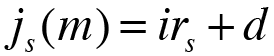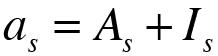The principal   C0 Number of years   n Number of shorter time units in one year   m Annual payment (1) Semiannual payments (2) Four-monthly payments (3) Quarterly payments (4) Bimonthly payments (6) Monthly payments (12) Weekly payments (52) Daily payments (365) Other: Margin or spread   d

Enter the corresponding rate of interest of each year

 Año 1 i1 =Annual effective rate of interestEffective rate of interest per time unitNominal rate of interest
Calculate more precisely

To obtain amortization payments ( as ) of a loan with constant principal repayments you have to calculate the principal or capital repayments ( As ) firstly, dividing the principal ( C0 ) by the number of periods:Once calculated the principal or capital repayments ( As ), which are equal at all periods, you have to calculate the rate of interest of each year. You have to take into account the index rate ( irs ) of each year has to be adjusted by the margin or spread ( d ), with the exception of the first year interest rate, which is not subject to this type of adjustment. The adjustment is calculated on the basis of the following formula:With the above formula you can obtain the nominal rate of interest of this period ( j(m) ) and you need the Effective rate of interest per time unit ( i(m) ), this is why you have to do the following conversion:Once calculated the rate of interest of eacj period, yo have to calculate the amortization payments ( as ) of each perido, with the following formula:The principal or capital repayments ( As ) have been calculated before and we know that they are equal at all periods, so you have to calculate the interest payments ( Is ) of each period with the following formula:Once obtained the interest payments ( Is ) you only have to add up the principal or capital repayments ( As ) in each period in order to obtain the amortization payments ( as ).